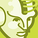New to Qlik Sense

If you’re new to Qlik Sense, start with this Discussion Board and get up-to-speed quickly.

Announcements
QLIKWORLD LIVE! MAY 16 - 19TH, EARLY BIRD DISCOUNTS! REGISTER TODAY
cancel
Showing results for
Did you mean:Contributor

Use variable as measure in set analysis

Hello all,

I've started with QLik sense a few months ago, today I'm stuck with a variable "issue".

I've read a lot of posts and suggestions but nothing help.

I have a variable called v_FTtotalcost, this variable is the sum of a dozen of other variables and is set as :

\$(=v_1) + \$(=v_2)+\$(=v_3)+\$(=v_4)+.... all are measures

(I've tried to build the v_FTtotalcost with Rangesum as well, but does help to solve my issue)

Want I want to achieve is to use v_FTtotalcost as measure in a set analysis to put a condition on the date .

I've tried many things as :

note that SUM(\$(=v_FTtotalcost)) gives a result (for all years of course..)

1 Solution

Accepted SolutionsMVP

@FabQlik wrote:

\$(=v_1) + \$(=v_2)+\$(=v_3)+\$(=v_4)+.... all are measures

How each variable calculates? If, It has Sum(Measure). It never replies with Sum({<>} Sum(Measure))

To achieve, you need to define with Aggr() like Sum({<>} Aggr(\$(Variable), Dim1, Dim2))

Before develop something, think If placed (The Right information | To the right people | At the Right time | In the Right place | With the Right context)
5 RepliesMVP

@FabQlik wrote:

\$(=v_1) + \$(=v_2)+\$(=v_3)+\$(=v_4)+.... all are measures

How each variable calculates? If, It has Sum(Measure). It never replies with Sum({<>} Sum(Measure))

To achieve, you need to define with Aggr() like Sum({<>} Aggr(\$(Variable), Dim1, Dim2))

Before develop something, think If placed (The Right information | To the right people | At the Right time | In the Right place | With the Right context)Contributor
Author

Indeed other variables are SUM, not familiar with Aggr function, I don't need any dim, what should I use as dim ?MVP

Can you tell us, What this variable? v_FTtotalcost

Make sure, Please write full expression at least 2 measures like Sum(V1)+Sum(V2) ...

Before develop something, think If placed (The Right information | To the right people | At the Right time | In the Right place | With the Right context)Contributor
Author

v_FTTotal is defined as :

\$(=v_Cost_Sales_Services) + \$(=v_Cost_Sales_Hardware) + \$(=v_Cost_NP_Services) + \$(=v_Cost_NP_Hardware) + \$(=v_SAC_NP_Services_Noweb) + \$(=v_SAC_NP_Hardware_Noweb) + \$(=v_SAC_NP_Hardware_Webonly) + \$(=v_SAC_ServicesNoWeb) + \$(=v_SAC_HardwareNoWeb) + \$(=v_SAC_HardwareWebonly)

Here is the definition of some v_Cost_xxxx  and v_SAC_xxx  variables (quite 'complex variables, with already some other variables included)

v_Cost_Sales_Hardware=

((SUM({\$< TM_PA_IND-={'Service'}, CONTR_STATUS_FAMILY= {'deactivation'},OPEN_AMT={">0"} >}OPEN_AMT))-(SUM({\$< TM_PA_IND-={'Service'}, CONTR_STATUS_FAMILY= {'deactivation'} >}CONTR_UNIQUE_CNT)*
(if(Action_Year='2018','190',if(Action_Year='2019','180')))))
*0.3

v_SAC_ServicesNoWeb=

SUM({\$< SA_MACRO_SEGMENT-={'TELE RES','WEBCHANNEL'}, TM_PA_IND={'Service'}, CONTR_STATUS_FAMILY= {'deactivation'} >}CONTR_UNIQUE_CNT)
* \$(v_SAC)
* (1-\$(v_Cback%ServiceNoWeb))

v_Cost_NP_Hardware=

(Sum({\$<TM_PA_IND-={'Service'}, CUSTOMER_BEHAVIOR_FLAG= {'NP deactivation'}>}NP_OPEN_AMT)
-
(Sum({\$<TM_PA_IND-={'Service'},CUSTOMER_BEHAVIOR_FLAG= {'NP deactivation'}>}CONTR_UNIQUE_CNT)
*
(if(Action_Year='2018','190',if(Action_Year='2019','180'))))
)
*0.3Contributor
Author

I 've succeeded to make it work thanks to your proposal : Sum({<>} Aggr(\$(Variable), Dim1, Dim2))

I put the Year as Dim , and I'm able to create a YTD condition in set analysis.

Solution :

Sum({< [ACTION_YYYYMM.autoCalendar.Year]= {"\$(=max(Action_Year))"}>}aggr(\$(v_FTTotal) , [Action_Date.autoCalendar.Year] ))

gives the v_FTtotal for max action year

Many ThxTags
Community Browser